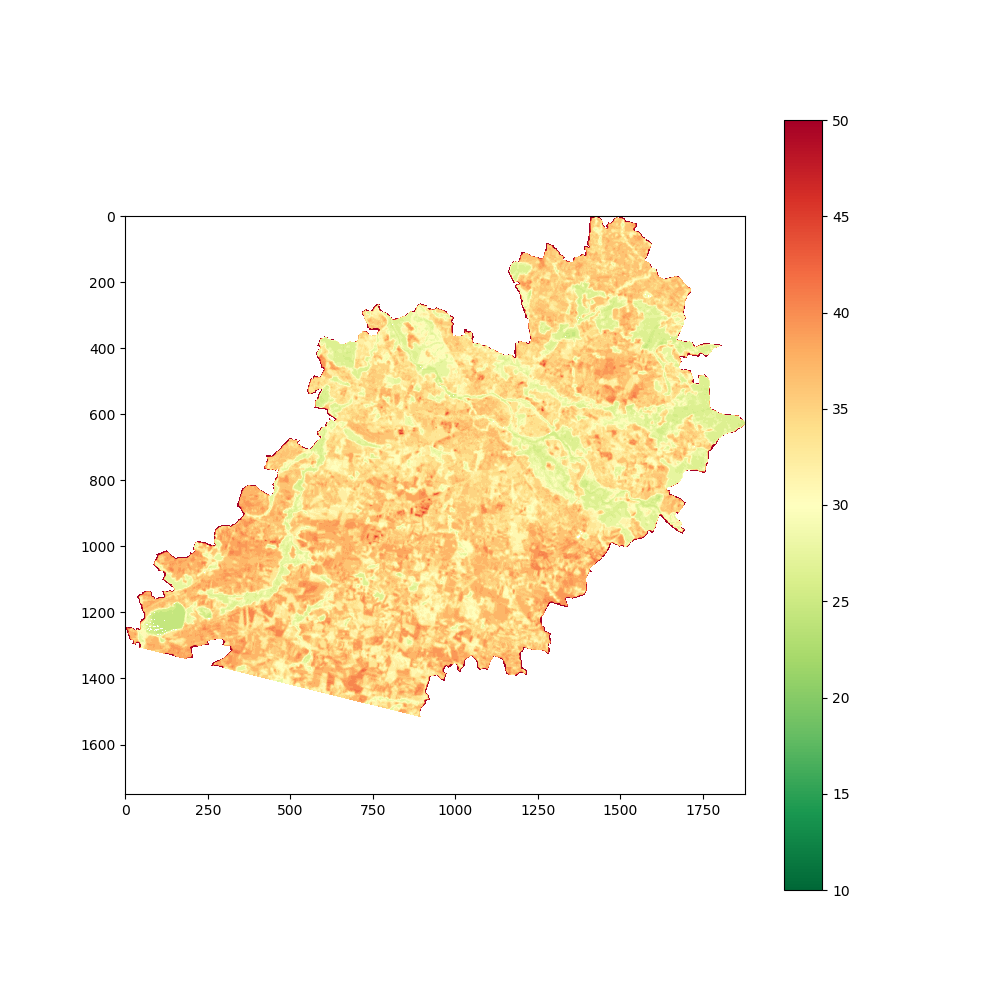Top
Sp.4ML > Data Engineering  > Toolbox: Visualize raster band in Python## Toolbox: Visualize raster band in Python

Toolbox notes are short and precise. There is no introduction. Just code and few comments about it. Article is designed to make it easy to reproduce the code and understand how it works.

## Script to read and show all rasters in the given directory

• Imported libraries: os, NumPy, Rasterio, matplotlib.
• Two functions: read_images() and show_band().
• read_images() takes two arguments: folder_name and file_end. Parameter folder_name is a string with a path to the directory which contains tiff rasters. Parameter file_end is used to differentiate useful data from the other files. This function is not covered in the note.
• show_band() takes four parameters. One is necessary and three are optional. Starting from the first… band is a numpy array read by the rasterio from the tiff file. It is processed inside the script to change all nan_val to numpy nan’s which are invisible in the matplotlib figure. (nan_val is the second parameter and its default value is zero). We shouldn’t perform this operation on the “living thing” to avoid problems with the data integrity and analysis output, so it is limited to the function scope.
• Parameter color_map is taken from the Matplotlib’s colormaps (https://matplotlib.org/3.1.0/tutorials/colors/colormaps.html). Default value is Set1 due to the fact that image in this example is a classification output and has only few numbers.
• The last parameter is title. It is a string which describes image content. If none is passed then image title doesn’t appear.
• The main function works as follow: variable images is a list with the paths to each ‘.tif’ file stored in the folder where script is invoked. Then each element from the list is read by rasterio. Pixels are passed into the show_band() function. Title is set to be a full path to the presented image. Parameter color_map is changed to ‘Accent’ because it gives better visual results than the default ‘Set1’ colormap.
import os
import numpy as np
import rasterio as rio
import matplotlib.pyplot as plt

file_list = os.listdir(folder_name)
channel_list = []
for f in file_list:
if f.endswith(file_end):
channel_list.append(folder_name + f)
channel_list.sort()
return channel_list

def show_band(band, nan_val=0, color_map='Set1', title=None):
band = band.astype(np.float)
band[band == nan_val] = np.nan
plt.figure(figsize=(11, 11))
image_layer = plt.imshow(band)
image_layer.set_cmap(color_map)
plt.colorbar()
if title is not None:
plt.title(title)
plt.axis('off')
plt.show()

if __name__ == '__main__':
file_ending = '.tif'
for img in images:
with rio.open(img) as src:
show_band(image, nan_val=0, color_map='Accent', title=img)

Output:##### Szymon
Subscribe
Notify of